# WBJEE Maths Test - 4

## 75 Questions MCQ Test WBJEE Sample Papers, Section Wise & Full Mock Tests | WBJEE Maths Test - 4

Description
Attempt WBJEE Maths Test - 4 | 75 questions in 120 minutes | Mock test for JEE preparation | Free important questions MCQ to study WBJEE Sample Papers, Section Wise & Full Mock Tests for JEE Exam | Download free PDF with solutions
QUESTION: 1

Solution:
QUESTION: 2

Solution:
QUESTION: 3

### If the co-efficients of rth and (r+1)th terms in the expansion of (3+7x)29 are equal, the r is equal to

Solution:
QUESTION: 4
If in the expansion of (1 + x)20, the coefficients of rth and (r + 4)th terms are equal, then r is
Solution:
QUESTION: 5

If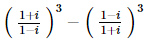= a + ib , then a and b are

Solution:
QUESTION: 6

Circles x2+y2-6x-2y+1=0 and x2+y2+2x-8y+13=0

Solution:
QUESTION: 7

For what values of x and y ,the complex numbers 9y2 - 4 - 10xi and 8y2 + 20i7 are conjugate to each other ?

Solution:
QUESTION: 8

The area bounded by two curves y2=4ax and x2=4ay is

Solution:

The curves y2 = 4ax and x2 = 4ay intersects at points where (x2/4a)2=4ax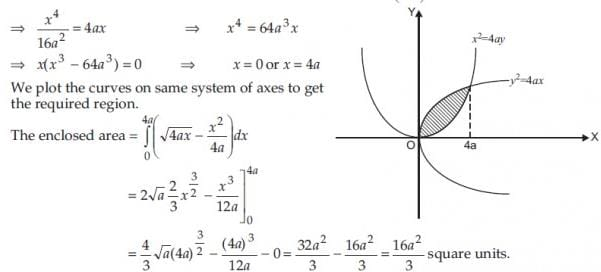QUESTION: 9

If a < 0 < b then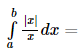Solution:
QUESTION: 10

If a ≠ 6, b, c satisfy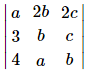= 0 then abc =

Solution:
QUESTION: 11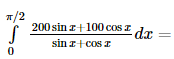Solution:
QUESTION: 12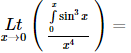Solution:
QUESTION: 13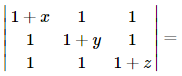Solution:
QUESTION: 14
The solution of the equation ydx-2xdy=0 gives parabolas whose common axis and the tangent at the vertex respectively, are :
Solution:
QUESTION: 15
The solution of the equation xdy-ydx=0 is
Solution:
QUESTION: 16

If f(x)=logx2log(x), then at x=e, f'(x)=

Solution:
QUESTION: 17

For real values of x the minimum value of [(1-x+x2)/(1+x+x2)] is

Solution:
QUESTION: 18

The eccentricity of the ellipse 9x2 + 5y2 − 30y = 0 is

Solution:
QUESTION: 19
If the two intersecting lines intersect the hyperbola and neither of them is a tangent to it, then number of intersecting points are
Solution: The general equation of a straight line is linear: ax + by + c = and the general equation of a hyperbola (but it's the same for all conics) is a second degree equation:
1. Ax2 + Bxy + Cy2 + Dx + Ey + F = 0
So, finding y ( or x ) from the first equation and substituting in the second, we have a second degree equation that has, at most, two real solutions. The possible cases are:
1) two real distinct solutions: the line is secant at two points
2) two coincident solution: the line is tangent
3) no real solution : the line is e''external''
4) the equation reduce to a first degree equation so we have one real solution and we say that the other solution goes to infinity.
So, for two lines that are not tangent we can have:
no common point with the conic if the two lines are external,
2 points if one is secant at two pints and the other is external or if both have one real common point with the conic an the other at infinity,
3 common points if one is secant at two points and the other at one point,
4 common points if the two lines are both secants at two points.
QUESTION: 20

The eccentricity of the ellipse 4x2 + 9y2 + 8x + 36y + 4 = 0 is

Solution:
QUESTION: 21

If φ (x) = f (x) + f (1 − x) and f ″ (x) < 0 in − 1,1 , then φ (x) strictly increases in the interval

Solution: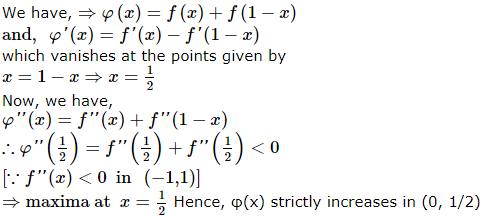QUESTION: 22

If sin⁻1x + sin⁻1y = 2π/3, then cos⁻1 x + cos⁻1 y is equal to

Solution:
QUESTION: 23

if f : R→R be such that f(1) = 4 and f'(1) = 12 then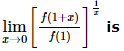Solution:
QUESTION: 24

If each element of a 3 x 3 matrix is multiplie by 3, then the determinant of the newly formed matrix is

Solution:
QUESTION: 25
The minimum value of f(x) = x4-x2-2x+6 is
Solution: f'(x) = 4x3 - 2x - 2
∴ f'(x) = 0 ⇒ 2x3 - x - 1 = 0 ⇒ (x - 1)(2x2 + 2x + 1) = 0
∴ x = 1.
Now, f" (x) = 12x 2 - 2 ∴ f" (1) = 12 - 2 > 0.
Hence, global min f(x) = 1 - 1 - 2 + 6 = 4.
QUESTION: 26
The HCF of 155 and 1385 is
Solution:
QUESTION: 27

The focus of the parabola x2-8x+2y+7=0 is

Solution:
QUESTION: 28

The tangents at the points (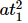,2at1) , (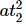, 2at2) on the parabola y2 = 4ax are at right angles if

Solution:

Tangent at t1 is yt1 = x +Tangent at t2 is yt2 = x +Tangents are at right angles
⇒ t1t2 = - 1

QUESTION: 29
In how many different ways can the letters of the word BOOKLET be arranged such that B and T always come together?
Solution:
QUESTION: 30

The equation 16x2 + y2 + 8xy − 74 x − 78y + 212 = 0 represents

Solution:
QUESTION: 31

The numbers of all words formed from the letters of the word CALCUTTA is

Solution:

No of letter in the given word is 8
No of repeated word is "C" 2 times, "A" 2 times and "T" 2 times. So the equation is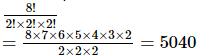QUESTION: 32

If A, B, C are three mutually exclusive and exhaustive events of a trial such that P(A) = 2P(B) = 3P(C) then P(A) =

Solution:
QUESTION: 33

The number of 4-digit even numbers that can be formed using 0, 1, 2, 3, 4, 5, 6 without repetition is

Solution:

Each even number must have 0, 2, 4 or 6 in is units place
Here total number of digits = 7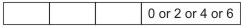When 0 occurs at units place there is no restriction on other places and when 2 or 4 occurs at units place there is restriction on thousands' place as 0 can not be put at thousands' place
Case I When 0 occurs at units place: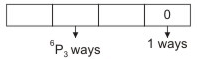∴ The number of numbers formed in this case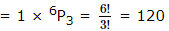Case II. When 0 does not occur at units place: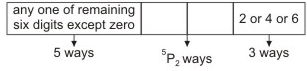The units' place can be filled up by any one of the three digits 2, 4 and 6 in 3 ways
∴ The number of numbers formed in this case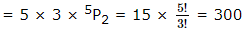∴ The required number = 120 + 300 = 420

QUESTION: 34
If A and B are two independent events in a sample space, then P(A̅/B̅) is equal to
Solution:
QUESTION: 35

The probability of a problem being solved by two students are 1/2 and 1/3 . The probability of the problem being solved is

Solution:
QUESTION: 36

The diameter of the circumcircle of a triangle with sides 5 cm, 6 cm and 7 cm is

Solution:
QUESTION: 37

Let |x| and [x] denote the fractional and integral part of a real number x respectively. Solution of 4 |x|=x+[x] are

Solution:
QUESTION: 38

If the ratio of the roots of the equation x2 + px + q = 0 be same as that of the roots of the equation x2 + lx + m = 0 then

Solution:
QUESTION: 39

If f(x) and g(x) are differentiable functions for 0≤x≤1 such that f(0) = 2, g(0) = 0, f(1) = 6, g(1) = 2, then in the interval (0,1),

Solution: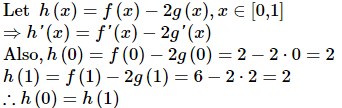Since f(x) and g(x) are differentiable in [0,1], h(x) is also differentiable in [0,1]. Hence, h(x) is also continuous in [0,1].So, all the conditions of Rolle's theorem are satisfied. Hence, there exists a point c, 0 < c < 1 for which h'(c) = 0.
∴ f ′ c − 2 g ′ c = 0, i . e . , f ′ c = 2 g ′ c

QUESTION: 40

In a ΔABC ,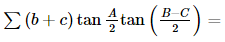Solution:QUESTION: 41

if the 10th term of a G.P.is 9 and 4th term is 4, then its 7th term is

Solution:

The terms of a G.P. are of form a, ar, ar2, ar3 ,.... ar9 = 9
So, r9 = 9 a .....(1)
ar3 = 4 .....(2)
Cubing both sides of (2), we get,
a3r9 = 64
putting value of r9 in this, we get,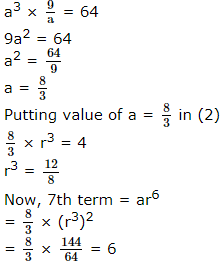QUESTION: 42

The sum of the series 1 + 1.3/6 + 1.3.5/6.8 + ....∞ is

Solution:
QUESTION: 43

((1)/(1 x 2)) + ((1/2) x (3)) + ((1/3) x (4)) + ... + ((1)/(n(n + 1))) equals

Solution:
QUESTION: 44
If the sum of first n positive intergers is 1/5 times the sum of their squares, then n equals :
Solution:
QUESTION: 45

The quadratic function f (x) whose graph goes through the points (-1, 4), (1, 0) and (2, 1) is

Solution:
QUESTION: 46

Which of the following is the empty set?

Solution:
QUESTION: 47

A points moves in such a way that the square of its distance from point(3,-2) is equal to numerically its distance from the line 5x-12y=13. The equation of the locus of the point is

Solution:
QUESTION: 48
Two vertices of a triangle are (4,-3) and (-2,5) and its ortho centre is (1,2), then its third vertex is
Solution:
QUESTION: 49

If the roots of the equation mx2 - 4x +2 (m+1)=0 are real, then

Solution:

Roots are real
⇒ discriminant ≥ 0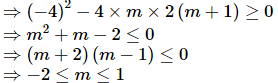QUESTION: 50

If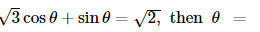Solution:
QUESTION: 51

Let n be a positive integer with f x = 1 ! + 2 ! + 3 ! + … + x ! and P x , Q x be polynomials in x such that
f x + 2 = P x f x + 1 + Q x f x ∀ x ≥ 1

Solution:
QUESTION: 52
In a triangle ABC with fixed base BC, the vertex A moves such that
$\mathrm{cos}B+\mathrm{cos}C=4\mathrm{sin}{}^{2}\frac{A}{2}$.
If a, b and c denote the lengths of the sides of the triangle opposite to the angles A, B and C, respectively, then
Solution:
QUESTION: 53

From a point p on the circle shown with centre O, the chord PA = 8cm is drawn. The radius of the circle is 24 cm let PB be drawn parallel to OA. Suppose BO extended meet PA extended at M. The length of MA equals (in cm).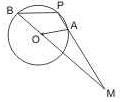Solution:
QUESTION: 54

The area bounded by the curves f (x) = sin−1 (sin x) and g (x) = [sin−1 (sin x) ] in the interval [0 ,π] , where [.] is a greatest integer function, is

Solution:
QUESTION: 55

The vertices A and C of a square ABCD are 2 + 3i and 3 − 2i respectively, then the vertices B and D are given by

Solution:
QUESTION: 56

Let f be real valued function such that f (2) = 2 and f ′ (2) = 1 , then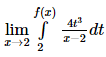equals

Solution:
QUESTION: 57

The value of f (0) , so that the function f defined as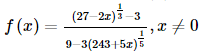is continuous at x = 0, is given by

Solution:
QUESTION: 58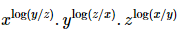equals

Solution:
QUESTION: 59

The ratio of the sum of 10th and 12th term of an AP are in the ratio 25 : 36. The ratio of the 31st term to 29th term can be

Solution:
QUESTION: 60

For any real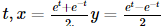is a point on the hyperbola x2 - y2 = 1. FInd the area bounded by this hyperbola and the line joining its centre to the points corresponding to t1 and -t1.

Solution:
QUESTION: 61

Let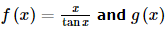and g x =xcosec x, where 0 < x ≤ 1 then in this interval

Solution:
QUESTION: 62

A hyperbola, having the transverse axis of length 2sin θ , is confocal with the ellipse 3x2 + 4y2 = 12. Then its equation is

Solution:
QUESTION: 63

If in triangle ABC , r1 > r2 > r3 then

Solution:
QUESTION: 64

Let f x = min(x, x2), for every real number x then,

Solution:
QUESTION: 65

The system of equations
3x − y + 4z = 3
x + 2y − 3z = − 2
6x + 5y + λz = − 3
has atleast one solution for

Solution:
QUESTION: 66

Area bounded by the curves y = 1 2 (2 − 3x − 2x2) below the line y = x + 1 and above the x-axis in square units is

Solution:
QUESTION: 67

In a triangle XYZ , ∠ Z =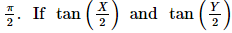are the roots of the equation ax2 + bx + c = 0, a ≠ 0 then

Solution:
*Multiple options can be correct
QUESTION: 68

Let cosA + cosB + cosC = 0 and sinA + sinB + sinC = 0, then which of the following statements is correct?

Solution:
QUESTION: 69

In a triangle ABC, tan C < 0. Then

Solution:
*Multiple options can be correct
QUESTION: 70

Let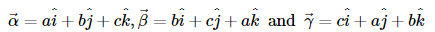be three coplanar vectors with a ≠ b , and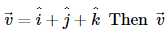is perpendicular to:

Solution:
*Multiple options can be correct
QUESTION: 71

Let z =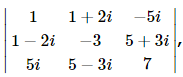, then:

Solution:
*Multiple options can be correct
QUESTION: 72

If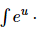sin 2x dx can be found in terms of known functions of x then u can be

Solution:
*Multiple options can be correct
QUESTION: 73

The equation of a common tangent to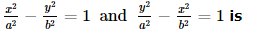Solution:
*Multiple options can be correct
QUESTION: 74

Let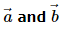be two non-collinear unit vectors. If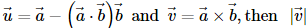is:

Solution:
*Multiple options can be correct
QUESTION: 75

If in a triangle ABC, CD is the angular bisector of the angle ACB then CD is equal to

Solution:Use Code STAYHOME200 and get INR 200 additional OFF Use Coupon Code